# How to Calculate ECN Commission

The commission rate depends on the account equity and the monthly trading volume. The commission rate can therefore decrease or increase from month to month depending on the traded volume in USD terms for previous period and also the account balance at the recalculation time.

The round-turn commission for positions with volume of 100,000 units of Base Currency (BC) or Underlying Asset (UA) is equal to 5 units (for round turn) of the base currency or underlying asset. Let us assume that  Equity  is in the  interval 1,000-5,000 and  Trade Volume  is less than 5,000,000 (50 lots).

### The detailed commission calculation for currency pairs

• where USD is the quote currency:

• Contract size = 100,000 BC units
• 1 unit of the BC = 1 GBP for GBP/USD, 1 EUR for EUR/USD etc.
• Commission = 5 units of the BC for the volume of 100,000 BC
• Traded volume (in units of BC) = 100,000 × V (in lots)
• Traded volume (in USD) = 100,000 × V (in lots) × Price

The definition implies, that commission in USD for the traded volume of 100,000 BC (1 lot) is:

5 BC units = 5 × Price (of the currency pair)

Let us assume that commission (in USD) for the traded volume V (in lots) is X (USD).

We can find out the value of X by solving the proportion: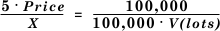So,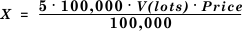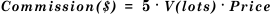or 0,005% of the traded volume in USD: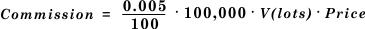where Price = the BID Price for SELL positions or the ASK Price for BUY Positions.

• where USD is the base currency:

• Contract size = 100,000 USD
• 1 unit of the base currency = 1 USD for USD/CHF, etc.
• Commission = 5 units of the BC for the traded volume of 100,000 BC
• Traded volume (in units of BC) = 100,000 × V (in lots)

The definition implies that commission in USD for the traded volume of 100 000 base currency units (1 lot) is 5 base currency units.

Let us assume that commission (in USD) for the traded volume V (in lots) is X (USD).

We can find out the value of X by solving the proportion: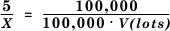So,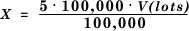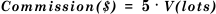or 0.005% of the traded volume in USD: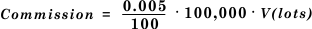where Price = the BID Price for SELL positions or the ASK Price for BUY Positions.

for crosses no USD (the base currency in the cross pair is quoted  directly against USD):

• Contract size = 100,000 base currency units
• 1 base currency unit = 1 GBP for GBP/JPY, 1 AUD for AUD/CAD etc.
• Commission = 5 base currency units for the volume of 100,000 base currency units
• Traded volume (in BC units) = 100,000 × V (in lots)
• Volume (in USD) = 100,000 × V (in lots) × Price (BC/USD)

The definition implies that commission in USD for the traded volume of 100,000 BC (1 lot) is:

5 BC units = 5 × Price (BC/USD)

Let us assume that commission (in USD) for the traded volume V (in lots) is X (USD).

We can find out the value of X by solving the proportion: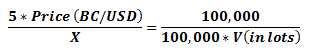So,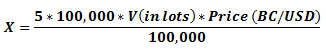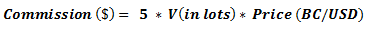or 0.005% of the traded volume in USD: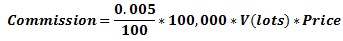where Price = BC/USD BID Price for SELL positions or BC/USD ASK Price for BUY Positions, when the base currency in the cross pair is quoted directly against USD.

for crosses no USD (the base currency in the cross pair is quoted against USD  indirectly):

• Contract size = 100,000 base currency units
• 1 base currency unit = 1 CAD for CAD/CHF, 1 CHF for CHF/GPY etc.
• Commission = 5 base currency units for the volume of 100,000 base currency units
• Traded volume (in BC units) = 100,000 × V (in lots)
• Volume (in USD) = 100,000 × V (in lots) ÷ Price (USD/BC)

The definition implies that commission in USD for the traded volume of 100,000 BC (1 lot) is:

5 BC units = 5 ÷ Price (USD/BC)

Let us assume that commission (in USD) for the traded volume V (in lots) is X (USD).

We can find out the value of X by solving the proportion: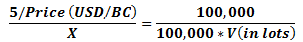So,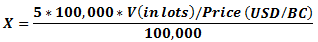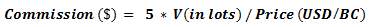or 0.005% of the traded volume in USD: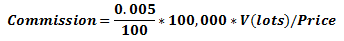where Price = USD/BC ASK Price for SELL positions or USD/BC BID Price for BUY Positions if the base currency in the cross pair is quoted against USD indirectly.

### The detailed commission calculation for metals

• Gold

• Contract size = 100 units of the underlying asset
• 1 unit of the underlying asset = 1 oz (troy ounce) for GOLD/USD
• 1 lot for GOLD/USD = 100 oz.
• Commission = 5 units of the underlying asset for the traded volume of 100,000 oz.
• Traded volume (in oz) = 100 (oz) × V (in lots)
• Traded Volume (in USD) = 100 (oz) × V (in lots) × Price

The definition implies that commission in USD for the traded volume of 100,000 units of the underlying asset (1,000 lots) is:

5 UA units = 5 (oz) × Price

Let us assume that commission (in USD) for the traded volume V (in lots) is X (USD).

We can find out the value of X by solving the proportion: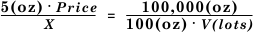So,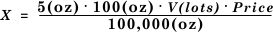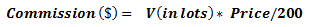or 0.005% of the traded volume in USD: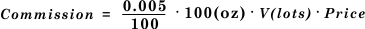where Price = the BID price for SELL positions, or the ASK price for BUY positions.

• Silver

• Contract size = 5,000 units of the underlying asset
• 1 unit of the underlying asset = 1 oz (troy ounce) for SILVER/USD
• 1 lot for SILEVR/USD = 5,000 oz.
• Commission = 5 units of the underlying asset for the traded volume of 100,000 oz.
• Traded volume (in oz) = 5,000 (oz) × V (in lots)
• Traded Volume (in USD) = 5,000 (oz) × V (in lots) × Price

The definition implies that commission in USD for the traded volume of 100,000 units of the underlying asset (20 lots) is:

5 UA units = 5 (oz) × Price

Let us assume that commission (in USD) for the traded volume V (in lots) is X (USD).

We can find out the value of X by solving the proportion: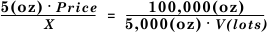So,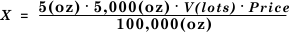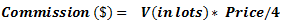or 0.005% of the traded volume in USD: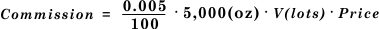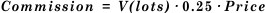• # Related Articles

• ## ECN

ECN stands for Electronic Communications Network formed by investment funds, banks, traders, etc. Due to the Forex market being largely unregulated, there is no central exchange for Forex and trading is performed on an OTC (Over The Counter) market. ...
• ## CFD - Commission

CFD trading has commission to open and to close a trade and the commission is deducted from Account Balance at the moment of opening the position. The commission for trades on commodities (XBRUSD, XTIUSD, XNGUSD) is calculated using the following ...
• ## Commission levels

Standard Commission coefficients The figure below shows the Standard Commission coefficients for the three agent types: Agent, Rebate I and Rebate II. Сoefficients are used in calculations of partner's remuneration. These are not final ...
• ## ECN Accounts

1. Are there any bonuses (promotions) in ECN accounts? No, we do not offer bonuses for ECN accounts 2. Are there any commissions in ECN accounts? ECN accounts have commissions to open/close a trade. The commission rate depends on the account equity ...
• ## Commission account

Multi-currency Commission account is intended for crediting partner remuneration and cashback. The balance of your Commission account is displayed on the left of the Client Portal. What is the difference between the Commission account and Main ...